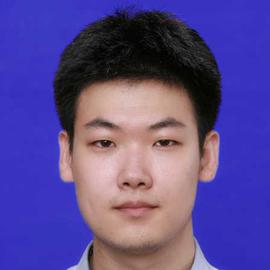# 第 20 次 CCF 计算机软件能力认证

## 点亮数字人生

### 题解

struct gate
{
int rev;
std::function<int(int, int)> aggr;
};


## 星际旅行

### 题解

$$d^2 = |OB|^2 - (\frac{\vec{OB} \cdot \vec{AB}}{|AB|})^2$$

$$|AB|^2(|OB|^2 - r^2) \geq (\vec{OB} \cdot \vec{AB})^2$$

$$\vec{AB} \cdot \vec{AO} < 0$$

$$\vec{BA} \cdot \vec{BO} < 0$$

$$|AP|^2 = |OA|^2 - r^2$$ $$|QB|^2 = |OB|^2 - r^2$$

$$cos^{-1} \left( \frac{\vec{OA} \cdot \vec{OB}}{|OA| \cdot |OB|} \right)$$

$$cos^{-1} \left( \frac{r}{|OA|} \right)$$

$$cos^{-1} \left( \frac{r}{|OB|} \right)$$

$$\angle{POQ} = \angle{AOB} - \angle{AOP} - \angle{BOQ}$$

## 密信与计数

### 问题重述

DFA 状态数 $n$ 不超过 $50$，$m$ 不超过 $1000$， 单词长度之和 $w$ 不超过 $50$。

### 题解

$10^8$ 复杂度跑 $93ms$ 真的离谱……##### Xi Ruoyao
###### PhD Student

Retired from ICPC, now a PhD student and an assistant ICPC coach (with no salary).